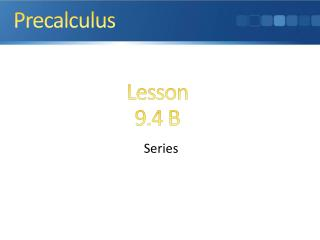DownloadDownload PresentationSeries

# Series

Download Presentation## Series

- - - - - - - - - - - - - - - - - - - - - - - - - - - E N D - - - - - - - - - - - - - - - - - - - - - - - - - - -
##### Presentation Transcript

1. Precalculus Lesson 9.4 B Series

2. Quick Review

3. What you’ll learn about • Summation Notation • Sums of Arithmetic and Geometric Sequences • Infinite Series • Convergences of Geometric Series … and why Infinite series are at the heart of integral calculus.

4. Summation Notation

5. Example Summation Notation

6. Example Summation Notation

7. Sum of a Finite Arithmetic Sequence

8. Example Summing the Terms of an Arithmetic Sequence

9. Sum of a Finite Geometric Sequence

10. Infinite Series

11. Sum of an Infinite Geometric Series

12. Example Repeating Decimals

13. Homework: Text pg747 Exercises # 28-58 even

14. Homework: Calculus Honors Text pg749 Exercises # 2-30 even